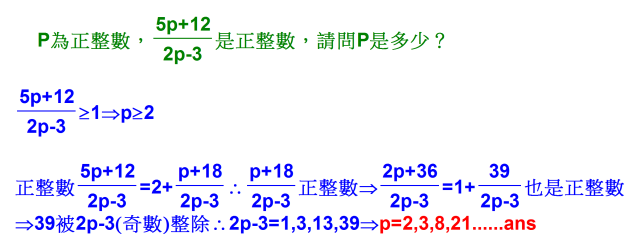## [國中]P為正整數，(5p+12)/(2p-3)=正整數，請問P是多少？### [國中]P為正整數，(5p+12)/(2p-3)=正整數，請問P是多少？

P為正整數，(5p+12)/(2p-3)=正整數，請問P是多少？

(5p+12)/(2p-3)
=[(4p-6)+(p+18)]/(2p-3)
=2+(p+18)/(2p-3) ←我不知道這個是甚麼算出來的？

cloriatw( 5P + 12 ) = ( 2P - 3 ) x n

5P + 12 = 2Pn - 3n

5P - 2Pn = - 3n - 12

( 5 - 2n ) P = -3 ( n + 4)

P = -3 ( n + 4 ) / ( 5 - 2n )

P = 3 ( n + 4 ) / ( 2n - 5 )

其中分母 2n - 5 必為奇數，

因為 P 也是正整數，所以分子 3 ( n + 4) 也必需要是奇數才行

而 3 為奇數，因此 ( n + 4 ) 也必為奇數，這樣乘起來才會是奇數！奇數才能跟奇數約分成整數！

故 n 必為奇數！

P = 3 ( n + 4 ) / ( 2n - 5 ) 為正整數

所以 n >2，然後再找 2 以上的奇數

n = 3 時，P = 3 ( 3 + 4 ) /  ( 6 - 5 )  ，得 P = 21

n = 5 時，P = 3 ( 5 + 4 ) / ( 10 - 5 ) ，得 P = 27 / 5

n = 7 時，P = 3 ( 7 + 4 ) / ( 14 - 5 ) ，得 P = 33 / 9

n = 9 時，P = 3 ( 9 + 4 ) / ( 18 - 5 ) ，得 P = 3

再往下，我有代到 n = 17，P 都沒有正整數，剩下的就請你自已試試吧！！devell